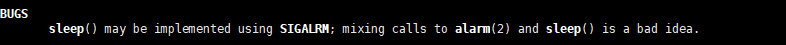# 如何实现限制上传或下载速度

## 预备知识

### 1.gettimeofday函数

#include<sys/time.h>

int gettimeofday(struct  timeval*tv,struct  timezone *tz )

gettimeofday()会把目前的时间用tv 结构体返回，当地时区的信息则放到tz所指的结构中。

timeval结构体定义为：

struct  timeval{
long  tv_sec;/*秒*/
long  tv_usec;/*微妙*/
}；

timezone结构体定义为：

struct  timezone{
int tz_minuteswest;/*和greenwich 时间差了多少分钟*/
int tz_dsttime;/*type of DST correction*/
}

### 2.nanosleep函数

#include <time.h>

int nanosleep(const struct timespec *req, struct timespec *rem);

struct timespec {
time_t tv_sec;        /* 秒 */
long   tv_nsec;       /* 纳秒 */
};


## 限速功能的实现

1.传输之前，先获取当前时间的秒数和微秒数并进行记录，每当传输1024字节之后，就判断当前的传输速度

	char buf;

//开始传输之前的时间
//获取当前时间的秒数和微秒数
sess->bw_transfer_start_sec = get_time_sec();
sess->bw_transfer_start_usec = get_time_usec();

//这是一个循环，所以能实现上传大文件
while (1) {
//从数据套接字接收数据
if (ret == -1) {
if (errno == EINTR) {
continue;
} else {
flag = 2;
break;
}
}
else if (ret == 0) {
flag = 0;
break;
}

//读取了一定数据之后需要判断是否限速
limit_rate(sess, ret, 1);
//睡醒之后判断是否收到了abor，如果是直接break,其实没有也可以，返回去
flag = 2;
break;
}

//写入文件中
if (writen(fd, buf, ret) != ret) {
flag = 1;
break;
}
}

2.判断是否超速，如果超速，进行休眠

//限速，计算睡眠时间，第二个参数是当前传输的字节数
void limit_rate(session_t *sess, int bytes_transfered, int is_upload)
{
//表明处于数据传输的状态
sess->data_process = 1;

// 睡眠时间=（当前传输速度/最大传输速度-1）*当前传输时间;
long curr_sec = get_time_sec();
long curr_usec = get_time_usec();

//流过的时间，当前所用的传输时间
double elapsed;
elapsed = (double)(curr_sec - sess->bw_transfer_start_sec);
//秒+微秒
elapsed += (double)(curr_usec - sess->bw_transfer_start_usec) / (double)1000000;
if (elapsed <= (double)0) {//等于0的情况有可能，因为传的太快了
elapsed = (double)0.01;
}

// 计算当前传输速度，传输的量除以传输时间,忽略了传输速度的小数部分
unsigned int bw_rate = (unsigned int)((double)bytes_transfered / elapsed);

double rate_ratio;
//上传
//当前速度小于上传速度
// 不需要限速，也需要更新时间
sess->bw_transfer_start_sec = curr_sec;
sess->bw_transfer_start_usec = curr_usec;
return;
}

//根据公式进行计算
}
//下载
else {
//不需要限速
sess->bw_transfer_start_sec = curr_sec;
sess->bw_transfer_start_usec = curr_usec;
return;
}

}

//计算睡眠时间
//睡眠时间=（当前传输速度/最大传输速度-1）*当前传输时间��;
double pause_time;
//需要睡眠的时间
pause_time = (rate_ratio - (double)1) * elapsed;

//进行睡眠
nano_sleep(pause_time);

//更新时间，下一次要开始传输的时间更新为当前时间
sess->bw_transfer_start_sec = get_time_sec();
sess->bw_transfer_start_usec = get_time_usec();

}

sleep函数中有说明：©️2019 CSDN 皮肤主题: 技术黑板 设计师: CSDN官方博客